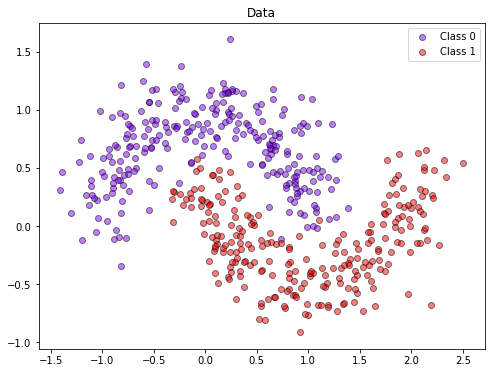# TensorFlow and Keras¶

In :
import numpy as np
import pylab as pl
from sklearn.datasets.samples_generator import make_moons

%matplotlib inline

# Functions for plotting 2D data and decision regions

def plot_data(X, y):
y_unique = np.unique(y)
colors = pl.cm.rainbow(np.linspace(0.0, 1.0, y_unique.size))
for this_y, color in zip(y_unique, colors):
this_X = X[y == this_y]
pl.scatter(this_X[:, 0], this_X[:, 1],  c=color,
alpha=0.5, edgecolor='k',
label="Class %s" % this_y)
pl.legend(loc="best")
pl.title("Data")

def plot_decision_region(X, pred_fun):
min_x = np.min(X[:, 0])
max_x = np.max(X[:, 0])
min_y = np.min(X[:, 1])
max_y = np.max(X[:, 1])
min_x = min_x - (max_x - min_x) * 0.05
max_x = max_x + (max_x - min_x) * 0.05
min_y = min_y - (max_y - min_y) * 0.05
max_y = max_y + (max_y - min_y) * 0.05
x_vals = np.linspace(min_x, max_x, 30)
y_vals = np.linspace(min_y, max_y, 30)
XX, YY = np.meshgrid(x_vals, y_vals)
grid_r, grid_c = XX.shape
ZZ = np.zeros((grid_r, grid_c))
for i in range(grid_r):
for j in range(grid_c):
ZZ[i, j] = pred_fun(XX[i, j], YY[i, j])
pl.contourf(XX, YY, ZZ, 30, cmap = pl.cm.coolwarm, vmin= 0, vmax=1)
pl.colorbar()
pl.xlabel("x")
pl.ylabel("y")


### 1. Multilayer neural network in TensorFlow¶

You need to create a neural network model in TF that is able to discriminate the two classes in the following dataset:

In :
X, Y = make_moons(n_samples=1000, noise= 0.2, random_state=3)
x_train = X[:500]
x_test  = X[500:]
y_train = Y[:500]
y_test  = Y[500:]

pl.figure(figsize=(8, 6))
plot_data(x_train, y_train)For this you will need to create a neural network with one hidden layer. You cannot use prebuilt models such as those in tf.estimator. Hint: extend the logistic regression example from the TensorFlow handout.